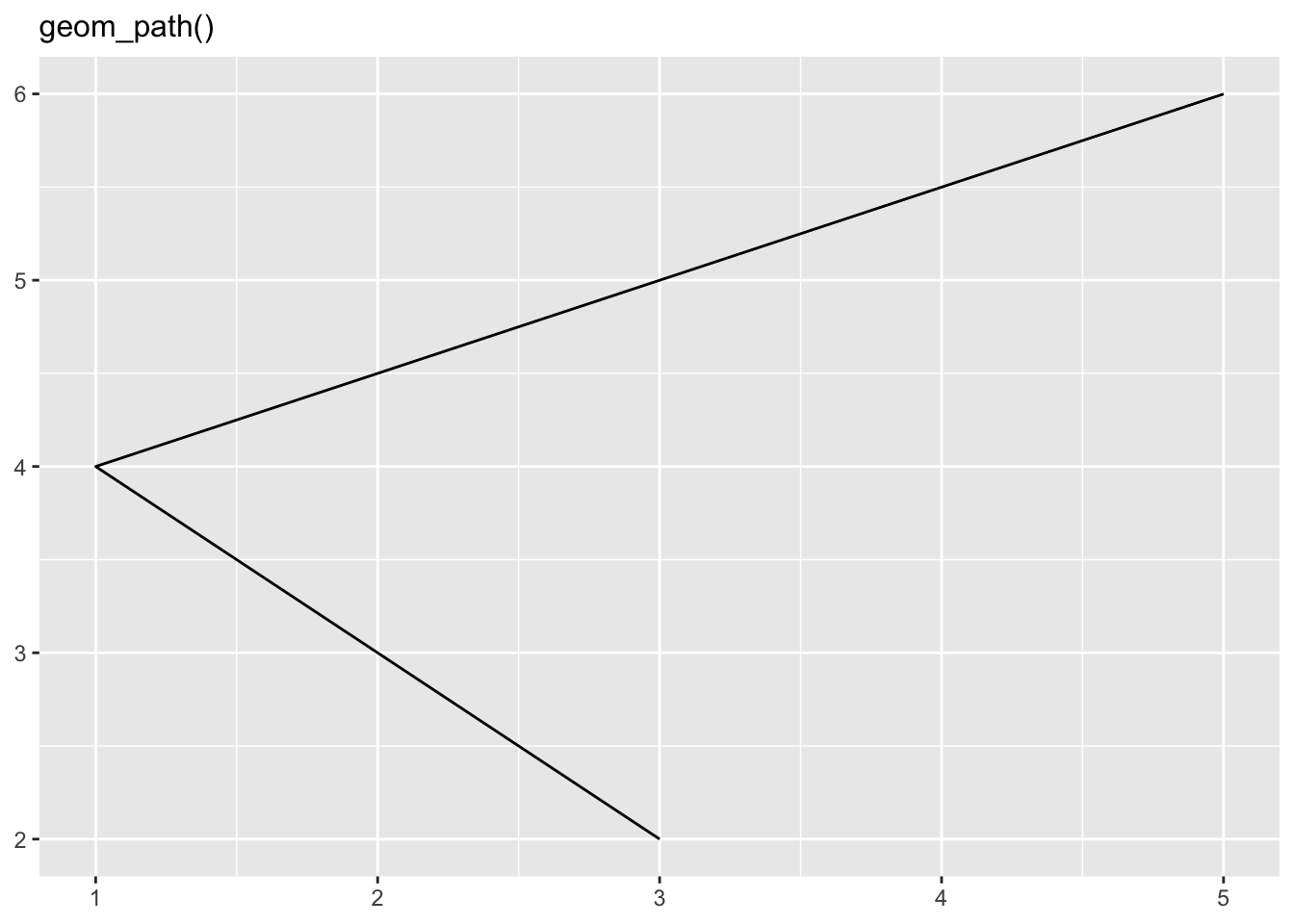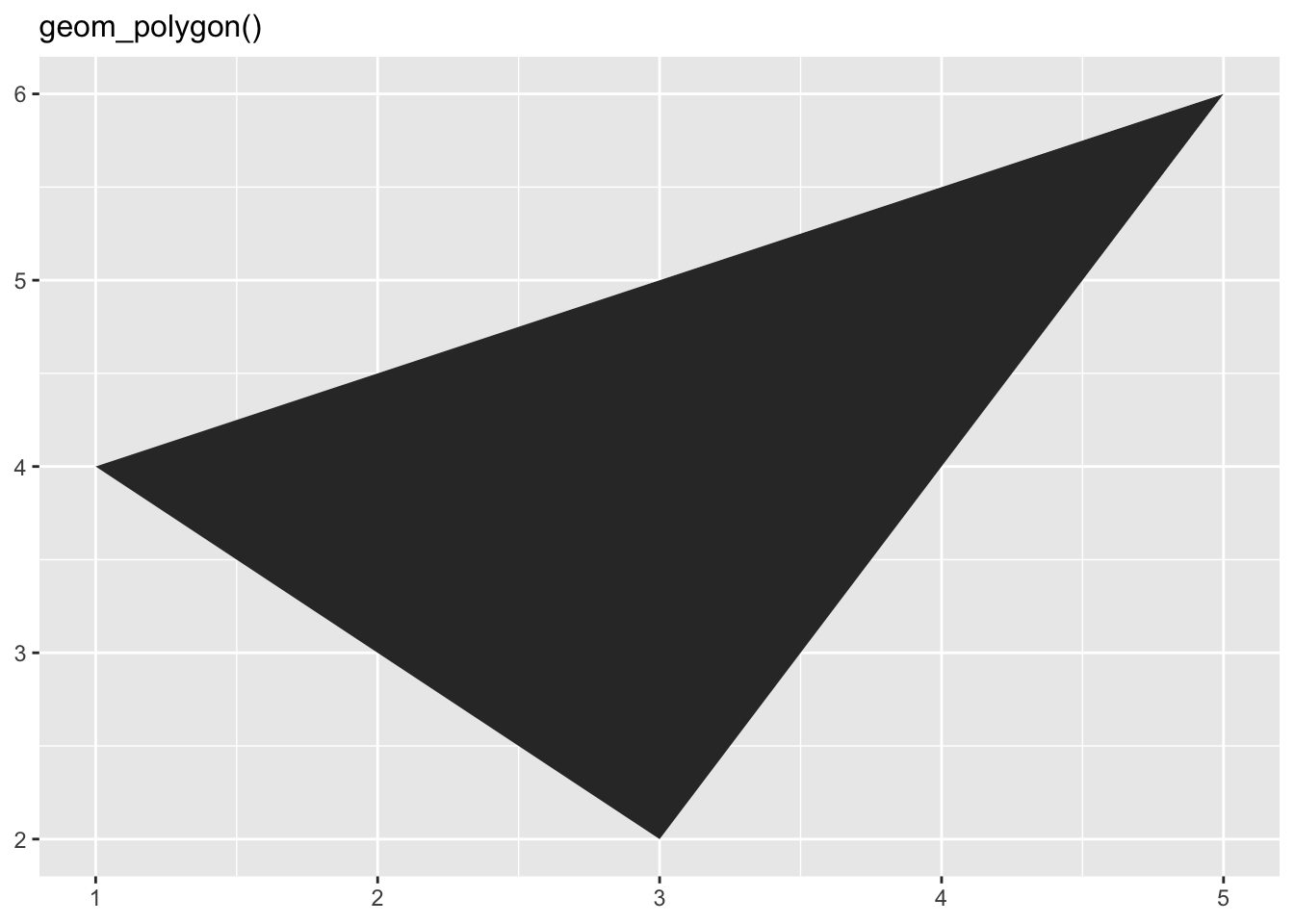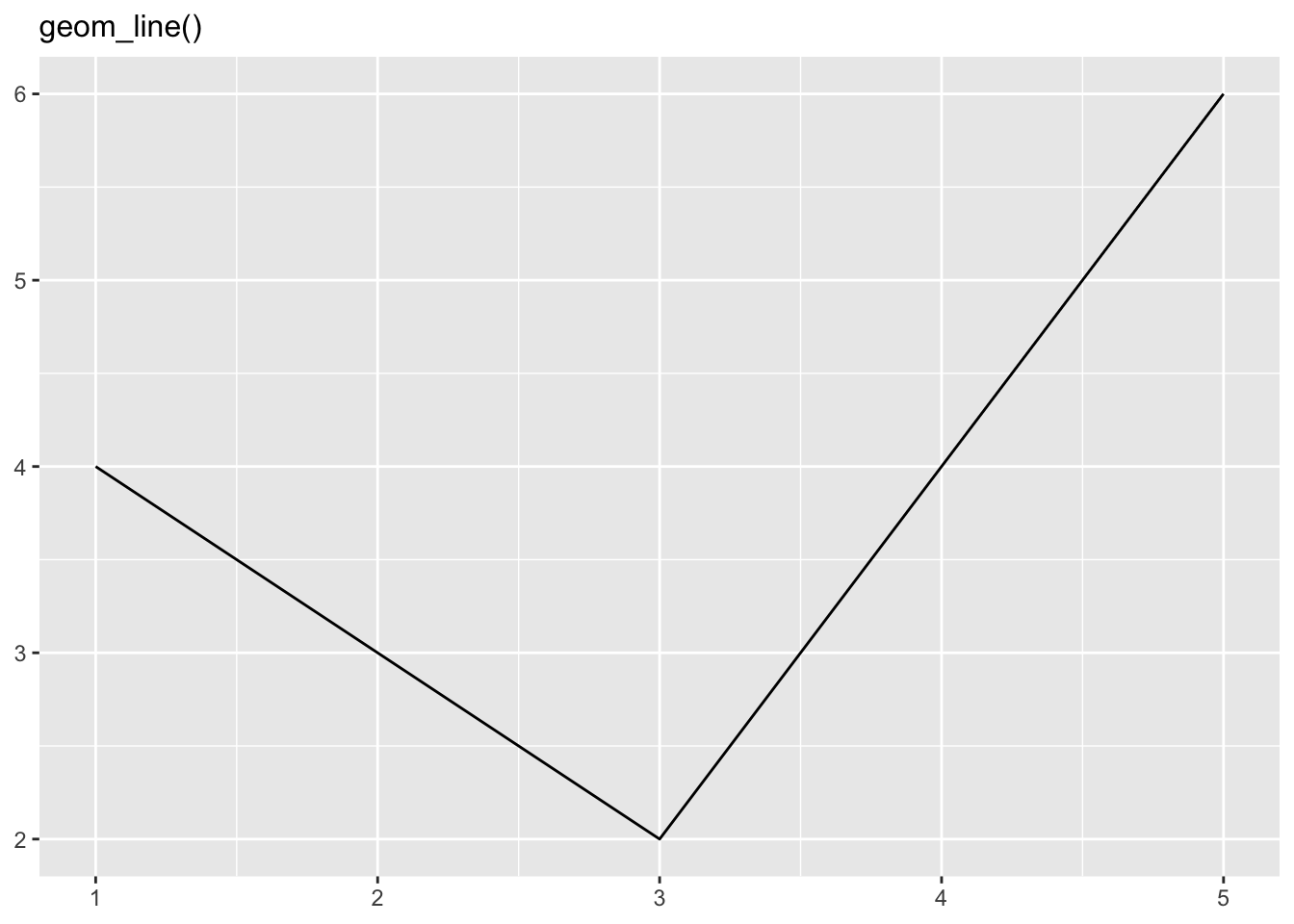# 3 Individual geoms

## 3.1 Exercises

1. What geoms would you use to draw each of the following named plots?

• Scatterplot: `geom_point()`
• Line chart: `geom_line()`
• Histogram: `geom_histogram()`
• Bar chart: `geom_bar()`
• Pie chart: ggplot2 does not have a geom to draw pie charts. One workaround, according to the R Graph Gallery is to build a stacked bar chart with one bar only using the `geom_bar()` function and then make it circular with `coord_polar()`

2. What’s the difference between `geom_path()` and `geom_polygon()`?

• `geom_polygon` draws the same graph (lines) as `geom_path`, but it fills these lines with color. See below:3. What’s the difference between `geom_path()` and `geom_line()`

`geom_line()` connects points from left to right, whereas `geom_path()` connects points in the order they appear in the data. See below:4. What low-level geoms are used to draw `geom_smooth()`? What about `geom_boxplot()` and `geom_violin()`?

• `geom_point()`, `geom_path()`, and `geom_area()` are used to draw `geom_smooth()`.
• `geom_rect()`, `geom_line()`, `geom_point()` are used for `geom_boxplot()`.
• `geom_area()` and `geom_path()` are used for `geom_violin()`